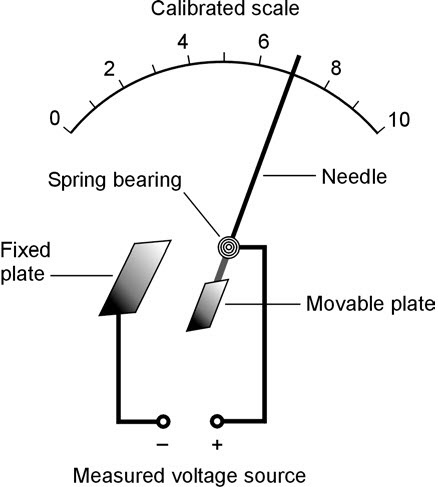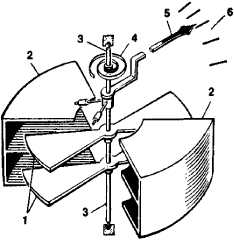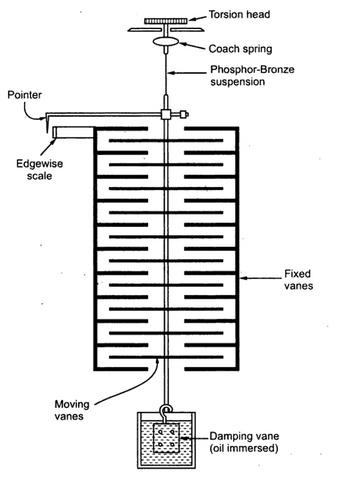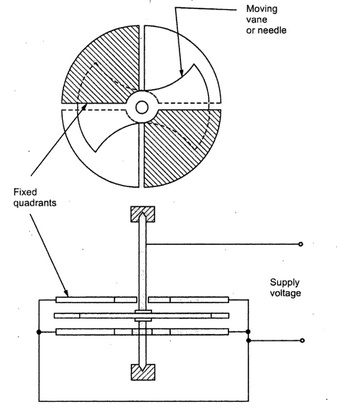# Measuring high voltages using Electrostatic Instruments

Electrostatic instruments are one of the different types of electromechanical indicating type instruments for the measurement of voltage, current or power. They can be used in both AC and DC measurement. AC measurement is only possible at one frequency. Most useful for high voltage device measurement where, only very little current can be supplied from the device that is being measured.

# Principle of working of Electrostatic instruments:

These types of devices work on the principle of attraction or repulsion between the static charges. The deflecting torque for the indicting instruments is given by the static charges developed between the electrodes. When two charged plate with opposite polarity are near each other, they store energy like a capacitor and there is a force of attraction between the two plates which tries to move the plates together. If one plate is fixed and the other moves but attached by a spring, the work done to move the plates together due to the attractive forces is proportional to the energy stored in them.

# Working of an electrostatic instrument:

## Linear motion

Consider two plates A and B where plate A is fixed while B is movable that is oppositely charged and plate B restrained by a spring connected to fixed point. With the force of attraction between the two plates at F newton and the capacitance between the two plates be C farad.The linear movement is given by the force of attraction between the plates which can be derived from its energy storage and the controlling torque is given by spring. Thus at steady state, the deflecting force = Controlling force and the displacement x of the plate is a function of square of the voltage, change in capacitance with change in displacement and spring constant.

Thus, the instrument has a non linear scale . Since it is independent on the current, the instrument is used to measure voltages of 20KV ( High Voltages)

## Circular motion.

Similar to the linear motion electrostatic instrument , the deflecting force is given by the force of attraction between two plates and the controlling torque is given by the spring.

Working similar to the rotational capacitors, the displacement is given as a function of angular displacement θ

Again the scale is non linear.# Types of Electrostatic instruments:

## Kelvin multicellular voltmeter:

This is based on linear motion of parallel electrodes. These devices have multiple plates of the capacitor in parallel.

Deflecting force - electrostatic attraction

Controlling force - the spring

Damping force - provided by oil.## Quadrant Voltmeter:

These devices are based on the circular motion electrostatic instruments. The have plates that are circular sized as a quadrant of a circle with a non linear scale.

Deflecting force - electrostatic attraction

Controlling force - the spring

Damping force - provided by oil.Diagonally opposite quadrants re fixed. A needle is suspended equidistant between the upper and lower quadrants making up a circular case. The voltage to be measured connected between the fixed quadrants that are connected together and the needle.

### Types of connection in quadrant voltmeter:

Heterostatic : External voltage source is used for instrument operation

A voltage higher than the measured voltage is given to the needle. The diagonally opposite vanes are connected in pairs. The needle is positively charged and the quadrants do not have any forces between them because it gets cancelled out. There is attractive and repulsive forces between the needle and the quadrants adjacent to each other.

Idiostatic : External voltage is not required for operation. Portable electrostatic instruments with air friction damping. Used in labs for the voltage measurement.

# Advantages of Electrostatic instruments

• Low power requirement

• No frequency error as heat generated is independent of frequency

• Stray magnetic field effect is absent

• Used I High voltage application

• Used for both AC and DC.

# Disadvantages of electrostatic instruments:

• Expensive

• Only used for high voltage measurement

• Non uniform scale

• Operating force is small.